# Kitten states via photon subtraction¶

In this tutorial we study how to prepare a cat state with a small amplitude (i.e. a kitten state) by doing photon subtraction. This idea goes back to the following paper: “Generating Schrödinger-cat-like states by means of conditional measurements on a beam splitter” Phys. Rev. A 55, 3184, (1997) by M. Dakna, T. Anhut, T. Opatrný, L. Knöll, and D.-G. Welsch.

:

import numpy as np
from qutip import wigner, Qobj, wigner_cmap

import matplotlib.pyplot as plt
import matplotlib as mpl
from matplotlib import cm

import strawberryfields as sf
from strawberryfields.ops import *
from thewalrus.quantum import state_vector, density_matrix


## Ideal preparation¶

Here we setup some basic parameters, like the value of the photon-number-resolving detectors we will use to herald and the amount of squeezing to use. We pick the squeezing parameter to be $$r = 1.0$$. We also fix the beam-splitter angle to have a high transmissivity (i.e. $$\theta \ll 1$$).

:

n = 1
r = -1.0
theta = np.arccos(np.sqrt(0.97))
theta

:

0.17408301063648038


Now we setup a 2-mode quantum circuit in Strawberry Fields and obtain the covariance matrix and vector of means of the Gaussian state

:

nmodes = 2
prog = sf.Program(nmodes)
eng = sf.Engine("gaussian")
with prog.context as q:
Sgate(r)|q
BSgate(theta,0.0)|q
state = eng.run(prog).state
mu = state.means()
cov = state.cov()

:

# Here we use the sf circuit drawer and standard linux utilities
# to generate an svg representing the circuit
file, _ = prog.draw_circuit()
filepdf = file[0:-3]+"pdf"
filepdf = filepdf.replace("circuit_tex/","")
filecrop = filepdf.replace(".pdf","-crop.pdf")
name = "kitten_circuit.svg"
!pdflatex  $file > /dev/null 2>&1 !pdfcrop$filepdf > /dev/null 2>&1
!pdf2svg $filecrop$name


Here is a graphical representation of the circuit. It is always assumed that the input is vacuum in all the modes.We can now inspect the covariance matrix and vector of means. Note that the vector of means is zero since we did not use any displacement gates in the circuit above.

:

print(np.round(mu,10))
print(np.round(cov,10))

[0. 0. 0. 0.]
[[ 1.19167168 -1.08989133 -0.          0.        ]
[-1.08989133  7.19738442  0.         -0.        ]
[-0.          0.          0.97406006  0.14750075]
[ 0.         -0.          0.14750075  0.16127522]]


We now use The Walrus to obtain the Fock representation of the photon-subtracted state when mode 0 is heralded in the value $$n=1$$ in the variable psi. We also calculate the probability of success in heralding in the variable p_psi.

:

cutoff = 20
psi = state_vector(mu, cov, post_select={0:n}, normalize=False, cutoff=cutoff)
p_psi = np.linalg.norm(psi)
psi = psi/p_psi
print("The probability of successful heralding is ", np.round(p_psi**2,5))

The probability of successful heralding is  0.03551


We now plot the photon-number distribution of the heralded state. Note that the state only has odd photon components,

:

plt.bar(np.arange(cutoff),np.abs(psi)**2)
plt.xlabel("$i$")
plt.ylabel(r"$p_i$")
plt.show()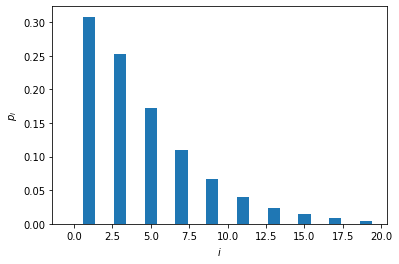We can now plot the Wigner function of the heralded state,

:

grid = 100
xvec = np.linspace(-5,5,grid)
Wp = wigner(Qobj(psi), xvec, xvec)
wmap = wigner_cmap(Wp)
sc1 = np.max(Wp)
nrm = mpl.colors.Normalize(-sc1, sc1)
fig, axes = plt.subplots(1, 1, figsize=(5, 4))
plt1 = axes.contourf(xvec, xvec, Wp, 60,  cmap=cm.RdBu, norm=nrm)
axes.contour(xvec, xvec, Wp, 60,  cmap=cm.RdBu, norm=nrm)
axes.set_title("Wigner function of the heralded state");
cb1 = fig.colorbar(plt1, ax=axes)
fig.tight_layout()
plt.show()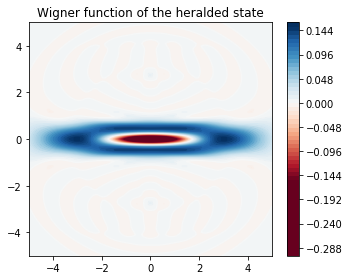and a cut of the Wigner function along $$p=0$$.

:

plt.plot(xvec, Wp[grid//2,:])
plt.title(r"$W(0,p)$")
plt.xlabel(r"p")
plt.show()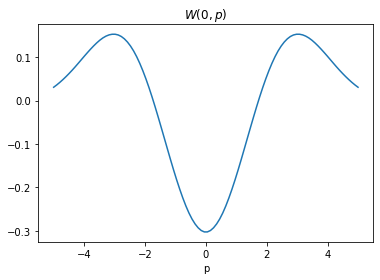We can now study what happens when loss in the heralding arm is increased. We will add loss in the heralding arm by varying the efficiency of the detector which is parametrized by a transmission $$\eta$$ ranging from $$\eta = 50\%$$ to $$\eta = 100\%$$ (ideal operation).

:

eta_vals = np.arange(1.,0.45,-0.05)
fidelities = np.zeros_like(eta_vals)
success_p = np.zeros_like(eta_vals)
nmodes = 2
for i,eta in enumerate(eta_vals):
prog = sf.Program(nmodes)
eng = sf.Engine("gaussian")
with prog.context as q:
Sgate(r)|q
BSgate(theta,0.0)|q
LossChannel(eta)|q
state = eng.run(prog).state
mu = state.means()
cov = state.cov()
rho = density_matrix(mu, cov, post_select={0:n}, normalize=False, cutoff=cutoff)
success_p[i] = np.real_if_close(np.trace(rho))
fidelities[i] = np.real_if_close(psi.conj() @ rho @ psi/success_p[i])


We now plot the probability of success of the heralding scheme as a function of the transmission.

:

plt.plot(eta_vals, success_p)
plt.xlabel(r"Transmission $\eta$")
plt.ylabel(r"Success probability")
plt.show()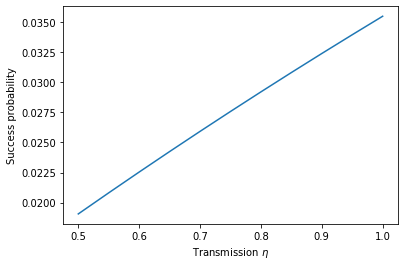and similarly study the fidelity of the heralded state with respect to the ideal (lossless) state.

:

plt.plot(eta_vals, fidelities)
plt.xlabel(r"Transmission $\eta$")
plt.ylabel(r"Fidelity with ideal state")
plt.show()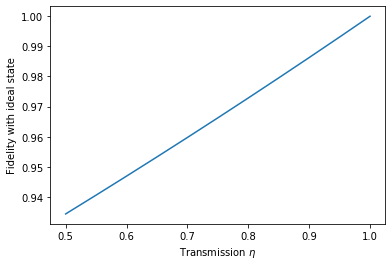We can also look at the photon number distribution of the nonideal state.

:

plt.bar(np.arange(cutoff),np.real_if_close(np.diag(rho/np.trace(rho))))
plt.xlabel(r"$i$")
plt.ylabel(r"$p_i$")
plt.show()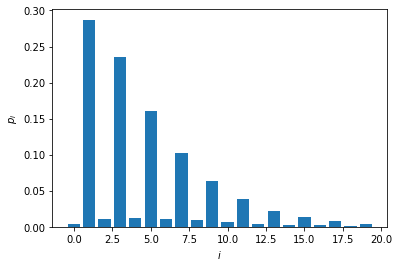Now we plot the Wigner function of the heralded state for $$\eta = 50\%$$.

:

sc1 = np.max(Wp)
W = wigner(Qobj(rho/np.trace(rho)), xvec, xvec)
nrm = mpl.colors.Normalize(-sc1, sc1)
fig, axes = plt.subplots(1, 2, figsize=(7.5, 3),sharey=True)
axes.contourf(xvec, xvec, Wp, 60,cmap=cm.RdBu, norm=nrm)
axes.contour(xvec, xvec, Wp, 60,  cmap=cm.RdBu, norm=nrm)
axes.set_title(r"$W(x,p)$ for $\eta = 1.0$");
cb1 = fig.colorbar(plt1, ax=axes)
plt2 = axes.contourf(xvec, xvec, W ,60, cmap=cm.RdBu, norm=nrm)
plt2 = axes.contour(xvec, xvec, W ,60, cmap=cm.RdBu, norm=nrm)
axes.set_title(r"$W(x,p)$ for $\eta = 0.5$");
cb2 = fig.colorbar(plt1, ax=axes)
fig.tight_layout()
plt.show()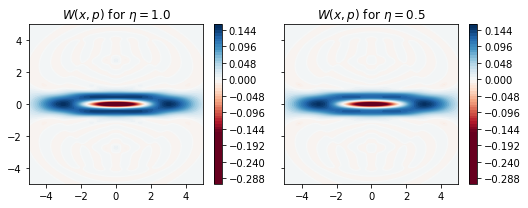and also a cut of the Wigner function along $$x=0$$.

:

plt.plot(xvec, W[grid//2,:], label=r"$\eta=0.5$")
plt.plot(xvec, Wp[grid//2,:], label=r"$\eta=1.0$")
plt.title(r"$W(0,p)$")
plt.xlabel(r"$p$")
plt.legend()
plt.show()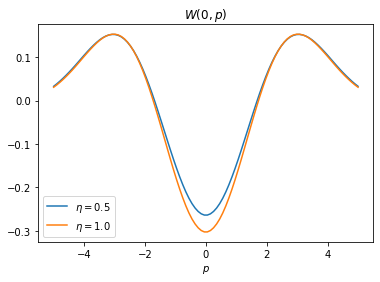Note that unlike for the case of a heralded Fock state, here loss in the heralding arms has very little effect. For a simple explanation of why this is the case cf. Sec. IV.B. of “Simulating realistic non-Gaussian state preparation” Phys. Rev. A 100, 022341 (2019) by N. Quesada, L. G. Helt, J. Izaac, J. M. Arrazola, R. Shahrokhshahi, C. R. Myers, and K. K. Sabapathy.

:

%reload_ext version_information
%version_information qutip, strawberryfields, thewalrus

:

SoftwareVersion
Python3.7.5 64bit [GCC 7.3.0]
IPython7.10.1
OSLinux 4.15.0 72 generic x86_64 with debian stretch sid
qutip4.4.1
strawberryfields0.13.0-dev
thewalrus0.11.0-dev
Mon Dec 30 10:12:11 2019 EST

Note

Click here to download this gallery page as an interactive Jupyter notebook.